Actions

# Calculation Module¶

## Generall¶

The calculation module is used to calculate loads or strains, as well as stresses within the specified laminate according to the classical laminated plate theory (,). In order to be able to directly output the effects of the laminate stacking sequence on the stiffness matrix, the loads or strains and the stresses of the layers, the calculations are updated automatically. Among other things, hygrothermal effects can also be taken into account .

During a calculation, the system of linear equations listed below is solved with the help of the Gaussian elimination method. The calculated unknowns are entered in the fields of the loads and strains. In addition, the stresses and strains in each laminate layer are calculated. These can be viewed in the internal forces subwindow in the local fiber coordinate system.

## Window Layout¶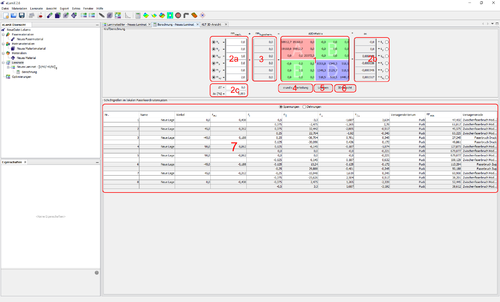### 1 - ABD matrix¶

Based on the stiffness matrices of the individual layers, the membrane stiffness matrix $\mathbf{A}$, coupling stiffness matrix $\mathbf{B}$ and bending stiffness matrix $\mathbf{D}$ of the laminate are automatically calculated with repect to the classical laminated plate theory and the three matrices are shown separately and color-coded within the ABD matrix.

Both membrane internal loads and moments as well as strain and curvatures can be specified as loads on the defined laminate. Either the load or the strain can be specified in the input fields under 2a and 2b for each coordinate direction. In addition, a temperature difference and the percentage change in the relative humidity can be specified in the fields in window section 2c. According to the classical laminated plate theory applies to mechanical and hygrothermal loads and strains

$$\begin{pmatrix} \underline{n} \\ \underline{m} \end{pmatrix}_{mech} = \begin{bmatrix} \mathbf{A} & \mathbf{B} \\ \mathbf{B} & \mathbf{D} \end{bmatrix} \begin{pmatrix} \underline {\varepsilon} \\ \underline {\kappa} \end{pmatrix} - \begin{pmatrix} \underline{n} \\ \underline{m} \end{pmatrix}_{hygrotherm}$$

If no effects of the hygrothermal loads are to be considered, the fields for the temperature difference and the percentage humidity difference must be filled with the values ​​zero. This view is the standard setting in the calculation module of eLamX².

### 3 - hygrothermal internal loads¶

At this point, the resulting hygrothermal internal loads are output based on the specified temperature difference and the percentage difference in humidity on the entire laminate. They are not input data. The selection of the check boxes for mechanical loads and the resulting distortions has no influence on the hygrothermal cutting loads. They result from the direction-dependent thermal conductivity and swelling coefficients of the individual layers as well as their stiffness matrices and the specified temperature and humidity difference.

### 4 - Stress and strain distribution button¶

This button can be used to display the stress and strain distribution within the laminate in local and global coordinates for the coordinate axes of the laminate plane. The output is only qualitative. The specified coordinate directions relate to the fiber angle, the specified x-value reflecting the size in the 0° direction and the y-value reflecting the size in the 90° direction of the laminate. The fiber orientations of the individual layers are shown by the hatching. Horizontal lines correspond to zero degrees and vertical lines to 90 degrees fiber angles. If the fiber angles of adjacent layers are very close together, the hatching is output in different colors.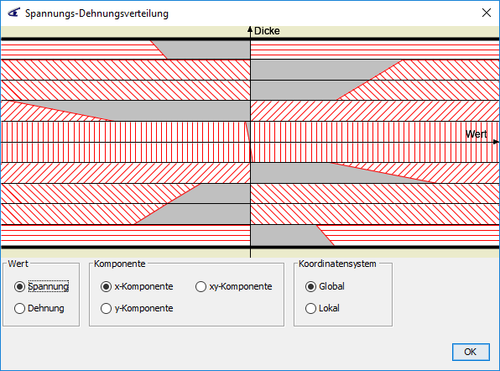### 5 - Delete button¶

Pressing the button deletes the specified loads and the calculated unknown internal loads and distortions, as well as the calculated stress distribution.

### 6 - Representation of the strains and curvatures on the basis of a square plate¶

This button can be used to open a window in which the calculated strains and curvatures are visualized on a square plate. This is used for a better understanding of the coupling effects within the ABD matrix.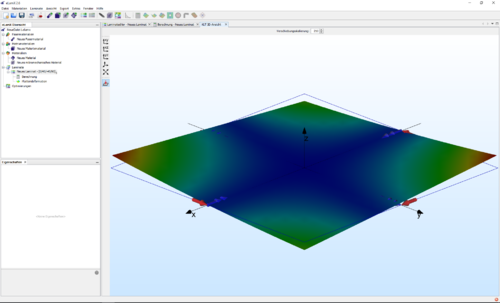### 7 - Layer data in the local fiber coordinate system¶

After a calculation, the stresses or strains of each layer of the composite are calculated in this part of the window, depending on the selection. The evaluation is carried out on the top and bottom of each individual layer. The stresses in each layer are compared with their strengths and a reserve factor is calculated on the top and bottom of the layer using the selected failure criterion. In addition, the type of failure to be expected is specified with regard to the failure criterion selected for each layer.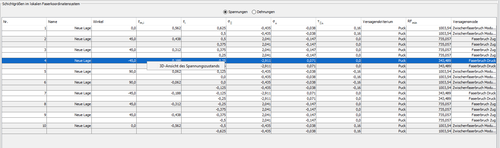In addition to displaying the failure criteria using the reserve factors, right-click on a layer to call up the Failure Criteria module and display the failure body of the individual layer together with the stress state in the stress area (see figure). The current stress on the composite layer is entered as a red point. If the calculated stress vector lies within the selected failure body, only its line is visible. This makes it clear which tension combination prevails in each layer.

## Laminate information / engineering constants¶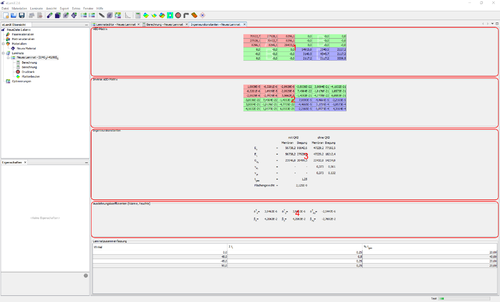This window can be opened by right-clicking on a laminate and then selecting engineering constants.

### 1 - ABD matrix¶

Based on the stiffness matrices of the individual layers, the membrane stiffness matrix $\mathbf{A}$, coupling stiffness matrix $\mathbf{B}$ and bending stiffness matrix $\mathbf{D}$ of the laminate are automatically calculated and color-coded when the calculation module is called up on the basis of classical laminated plate theory. This matrix is ​​shown in the form of a table so that all values ​​can be copied out.

### 2 - Compliance matrix of the laminate¶

The inverse of the stiffness matrix of the laminate is displayed here. It may be that for symmetrical laminates, terms of the inverse coupling stiffness matrix (b) are different from zero, but very small. This results from numerical inaccuracies in the double precision calculation within the program. This matrix is ​​shown in the form of a table so that all values ​​can be copied out.

### 3 - engineering constants of the laminate¶

The engineering constants of the laminate are also automatically calculated according to  for membrane and bending loads. This is done both with and without consideration of Poisson effect. In the case of asymmetrical laminates, where a coupling between membrane and bending deformation is present, the engineering constants have little meaning and should be used with caution.

### 4 - Coefficient of expansion of the laminate for heat and moisture¶

In this part of the window the thermal expansion coefficients $\alpha^T_i$ and the swelling coefficient $\beta_i$ of the total laminate are output according to the system of units that was selected for specifying the values ​​of the individual layer. Here, too, the definition according to  applies and thus
\begin{equation}
\left(
\begin{array}{c}
\epsilon_x \\
\epsilon_y \\
\gamma_{xy}
\end{array}
\right)_{T+H} =
\Delta T
\left(
\begin{array}{c}
\alpha^T_x \\
\alpha^T_y \\
\alpha^T_{xy}
\end{array}
\right) +
\Delta c
\left(
\begin{array}{c}
\beta_x \\
\beta_y \\
\beta_{xy}
\end{array}
\right)
\end{equation}

## Notes on the engineering constants¶

The engineering constants can be determined for two types of stress. On the one hand for a membrane stress and on the other hand for a bending stress. In both cases, this is possible with and without Poisson effect.

The approach is presented on the basis of the explanations in  for symmetrical laminates. For the membrane state, the ABD matrix becomes
\begin{equation}
\left(
\begin{array}{c}
n \\
m
\end{array}
\right) =
\left[
\begin{array}{cc}
A & B \\
B & D
\end{array}
\right]
\left(
\begin{array}{c}
\epsilon \\
\kappa
\end{array}
\right)
\end{equation}
only the $A$-matrix is ​​used, which is feasible for symmetrical laminates ($B = 0$). This results in
\begin{equation}
\left(
\begin{array}{c}
n_x \\
n_y \\
n_{xy}
\end{array}
\right) =
\left[
\begin{array}{ccc}
A_{11} & A_{12} & A_{13} \\
A_{21} & A_{22} & A_{23} \\
A_{31} & A_{32} & A_{33}
\end{array}
\right]
\left(
\begin{array}{c}
\epsilon_{x} \\
\epsilon_{y} \\
\gamma_{xy}
\end{array}
\right)
\end{equation}
The aim is now to come up with an equation for the stress $\sigma_{x}$, which corresponds to the Hooke's law $\sigma=E \epsilon$. To do this, the equation is divided by the total thickness of the laminate. This corresponds to a homogenization of the material and one obtains:
\begin{equation}
\left(
\begin{array}{c}
\sigma_x \\
\sigma_y \\
\tau_{xy}
\end{array}
\right) = \frac{1}{t_{ges}}
\left[
\begin{array}{ccc}
A_{11} & A_{12} & A_{13} \\
A_{21} & A_{22} & A_{23} \\
A_{31} & A_{32} & A_{33}
\end{array}
\right]
\left(
\begin{array}{c}
\epsilon_{x} \\
\epsilon_{y} \\
\gamma_{xy}
\end{array}
\right) \ .
\end{equation}
This equation corresponds to a single anisotropic layer across the entire thickness. There are two ways to come up with an equation according to the Hooke's law. On the one hand, $\epsilon_{y}$ and $\gamma_{xy}$ are set to zero without Poisson effect, and the following equations are obtained:
\begin{equation}
\left(
\begin{array}{c}
\sigma_x \\
\sigma_y \\
\tau_{xy}
\end{array}
\right) = \frac{1}{t_{ges}}
\left[
\begin{array}{ccc}
A_{11} & A_{12} & A_{13} \\
A_{21} & A_{22} & A_{23} \\
A_{31} & A_{32} & A_{33}
\end{array}
\right]
\left(
\begin{array}{c}
\epsilon_{x} \\
0 \\
0
\end{array}
\right) \ .
\end{equation}
\begin{equation}
\sigma_x = \frac{A_{11}}{t_{ges}}\epsilon_{x}
\end{equation}
In order to obtain a modulus of elasticity with Poisson effect, the equation has to be transformed and $\sigma_{y}$ and $\tau_{xy}$ are set to zero.
\begin{equation}
\left(
\begin{array}{c}
\epsilon_{x} \\
\epsilon_{y} \\
\gamma_{xy}
\end{array}
\right) = t_{ges}
\left[
\begin{array}{ccc}
A_{11} & A_{12} & A_{13} \\
A_{21} & A_{22} & A_{23} \\
A_{31} & A_{32} & A_{33}
\end{array}
\right]^{-1}
\left(
\begin{array}{c}
\sigma_x \\
0 \\
0
\end{array}
\right)
\ .
\end{equation}
\begin{equation}
\sigma_x = \frac{1}{(A^{-1})_{11} \cdot t_{ges}}\epsilon_{x}
\end{equation}
In this way, all engineering constants can now be determined. The constants with Poisson effect result from:
\begin{eqnarray}
E_x & = & \frac{1}{(A^{-1})_{11} \cdot t_{ges}} \\
E_y & = & \frac{1}{(A^{-1})_{22} \cdot t_{ges}} \\
G_{xy} & = & \frac{1}{(A^{-1})_{66} \cdot t_{ges}} \\
\nu_{xy} & = & -\frac{(A^{-1})_{12}}{(A^{-1})_{11}} \\
\nu_{yx} & = & -\frac{(A^{-1})_{12}}{(A^{-1})_{22}}
\end{eqnarray}
The constants without Poisson effect result from:
\begin{eqnarray}
E_x & = & \frac{A_{11}}{t_{ges}} \\
E_y & = & \frac{A_{22}}{t_{ges}} \\
G_{xy} & = & \frac{A_{66}}{t_{ges}} \\
\end{eqnarray}

If the Poisson effect is not taken into account, Poisson's ratios are not useful and are therefore not included.

These homogenized material parameters are only feasible for membrane loads. An equivalent procedure using the D-matrix is ​​necessary for bending, since the position of the layers has a great influence in the case of bending stresses in contrast to membrane stresses. The derivation of the formulas is taken from .

The analogy to the beam is used for bending. Since the laminate board has a rectangular cross-section, it is compared with the bending elasticity law of a rectangular beam and the following applies:
\begin{equation}
M = -EI \cdot w'' = - E \frac{b t^3}{12} \cdot w'' \rightarrow m = -E \frac{t^3}{12} \cdot w'' \ .
\end{equation}

In addition, $w'' = -\kappa$ applies.

Bending loads with the $D$-matrix are described for the laminate.
\begin{equation}
\left(
\begin{array}{c}
m_x \\
m_y \\
m_{xy}
\end{array}
\right) =
\left[
\begin{array}{ccc}
D_{11} & D_{12} & D_{13} \\
D_{21} & D_{22} & D_{23} \\
D_{31} & D_{32} & D_{33}
\end{array}
\right]
\left(
\begin{array}{c}
\kappa_{x} \\
\kappa_{y} \\
\kappa_{xy}
\end{array}
\right)
\end{equation}
Now the approach is equivalent to stressing the membrane. Assuming no Poisson effect results in:
\begin{equation}
\left(
\begin{array}{c}
m_x \\
m_y \\
m_{xy}
\end{array}
\right) =
\left[
\begin{array}{ccc}
D_{11} & D_{12} & D_{13} \\
D_{21} & D_{22} & D_{23} \\
D_{31} & D_{32} & D_{33}
\end{array}
\right]
\left(
\begin{array}{c}
\kappa_{x} \\
0 \\
0
\end{array}
\right)
\end{equation}
\begin{equation}
m_x = D_{11} \kappa_x
\end{equation}
\begin{equation}
E_{x,b} = \frac{12}{t_{ges}^3}D_{11}
\end{equation}

Thus, all engineering constants without Poisson effect result in:
\begin{eqnarray}
E_{x,b} & = & \frac{12}{t_{ges}^3}D_{11} \\
E_{y,b} & = & \frac{12}{t_{ges}^3}D_{22} \\
G_{x,b} & = & \frac{12}{t_{ges}^3}D_{66} \\
\end{eqnarray}
and with Poisson effect:
\begin{eqnarray}
E_{x,b} & = & \frac{12}{(D^{-1})_{11} \cdot t_{ges}^3} \\
E_{y,b} & = & \frac{12}{(D^{-1})_{22} \cdot t_{ges}^3} \\
G_{x,b} & = & \frac{12}{(D^{-1})_{66} \cdot t_{ges}^3} \\
\end{eqnarray}

At this point, too, Poisson's ratios are not very useful.

This approach generally only applies to symmetrical laminates. In the case of asymmetrical laminates, the inverse of the entire $ABD$ matrix must be formed and then the corresponding terms must be used.

 J.N. Reddy.
Mechanics of Laminated Composite Plates and Shells- Theory and Analysis.
CRC Press, Boca Raton, 2. auflage edition, 2003.
[BibTeX] [more]

 H. Schürmann.
Konstruieren mit Faser-Kunststoff-Verbunden.
Springer, 2004.
[BibTeX] [more]

 M. Barth.
Umsetzung eines Moduls zur Betrachtung von Temperatur und Feuchtigkeit in Faser-Kunststoff-Verbunden für das Programm eLamX.
ILR –LFT G 08-27, Technische Universität Dresden, April 2009.
[BibTeX] [more]

 H. Schürmann.
Konstruieren mit Faser-Kunststoff-Verbunden.
Springer, 2004.
[BibTeX] [more]

 H. Schürmann.
Konstruieren mit Faser-Kunststoff-Verbunden.
Springer, 2004.
[BibTeX] [more]

 H. Schürmann.
Konstruieren mit Faser-Kunststoff-Verbunden.
Springer, 2004.
[BibTeX] [more]

 H. Schürmann.
Konstruieren mit Faser-Kunststoff-Verbunden.
Springer, 2004.
[BibTeX] [more]

Updated by Andreas Hauffe about 2 years ago · 14 revisions